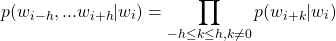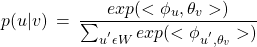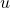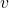# What is negative sampling when training the skip-gram model ?

Recap: Skip-Gram model is a popular algorithm to train word embeddings such as word2vec. It tries to represent each word in a large text as a lower dimensional vector in a space of K dimensions such that similar words are closer to each other. This is achieved by training a feed-forward network where we try to predict the context words given a specific word, i.e.,modelled aswhereis the context word andis a specific word

Why is it slow:  In this architecture, a soft-max function(above expression on RHS) is used to predict each context word. In practice, soft-max function is very slow in computation, especially for large vocabulary size.

Resolution :

• The objective function is reconstructed to treat the problem as a classification problem where pairs of words and its context form single training example. A given word and the corresponding context word are included in positive examples and a given word with non-context words are negative examples.
• While there can be a limited number of positive examples, there are many negative examples. Hence a randomly sampled set of negative examples are taken for each word when crafting the objective function.

This algorithm/model is called Skip Gram Negative Sampling(SGNS)